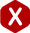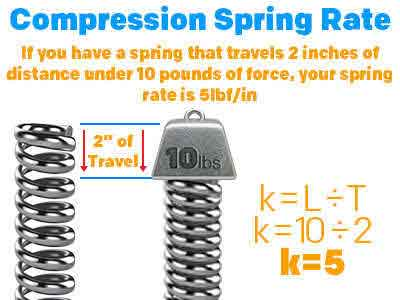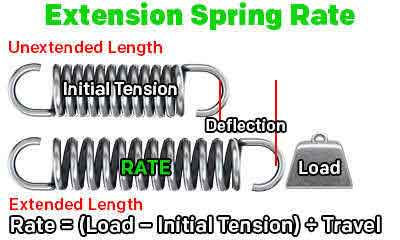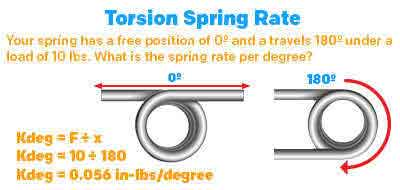<# Meaning of Spring Rate

## What Does Spring Rate Mean?

Definition: Spring rate is the proportion of a spring’s force (pounds or newtons) to one unit of travel (inch or millimeter). It is a constant value which helps you calculate how much load you will need with a specific distance traveled and it will also help you calculate how much travel you will achieve with a certain load.

Spring rate is a value measured in either pounds per inch (in the royal system) or newtons per millimeter (in the metric system). This value determines how much load you will be required to place on your spring in order to travel 1 inch of deflection. In any case your spring’s free length is smaller than 1 inch, keep in mind that this value is based on proportion. Meaning that if you have a spring which measures 0.250” and you want it to travel 0.050” with a spring rate of 15 lb/in, you must multiply the rate by the travel to get a total of 0.75 lbs. This means that with a rate of 15 lb/in, you will get 0.75 pounds of force for 0.05” of travel.

The previous calculation we did was to calculate the load on a spring based on spring rate and travel but, we may inverse the equation so that we may calculate the amount of spring rate we will need in order to accomplish certain working loads. If we convert the previous dimensions to the metric units most commonly used in the industry, we will convert the load from 0.75 pounds to 3.336 Newtons and the distance traveled of 0.05 inches to 1.27 millimeters. Then, we will divide the load by the distance traveled. This formula will give us a required spring rate of 15 pounds of force per inch.

Now, we will calculate the distance your spring will deflect or travel by taking the values in inches from the first calculation. We will divide the load of 0.75 pounds by the spring rate of 15 pounds per inch. The result to this calculation gives us a distance traveled of 0.05 inches. The formulas will be the same in metric units as well because the only thing changing are the units of measurement. Since this is a mathematical equation, the important factor are really the numbers.

### Extension Spring Rate

In the case of an extension spring, initial tension must be taken into the equation. This is an additional force besides the one considered in the spring rate. To calculate the required load, you will first calculate the rate and then add the initial tension. The result will be the required load in order to reach a certain extended length. In the case of spring rate, you must subtract the initial tension from the total load and then divide the result by the distance traveled. To calculate distance traveled, you will also subtract the initial tension from the load and then divide the result by the spring rate. Click on the link to learn more about initial tension.

### Torsion Spring Rate

For torsional springs, the same formulas you used at first will apply except these springs exert a radial force and not a linear one like compression and extension springs do. The only thing that would change in this case are the units. The torsion spring rate units are inch-pounds per degree (in-lbs/deg) and millimeter-Newtons per degree (mm-N/deg).